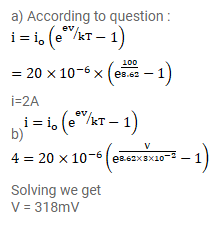# Consider a p-n junction diode having the characteristic

Question:

Consider a p-n junction diode having the characteristic $\mathrm{i}=\mathrm{i}_{0}\left(\mathrm{e}^{\mathrm{ev} / \mathrm{kT}-1)}\right.$ Where $\mathrm{i}_{0}=20 \mu \mathrm{A}$. The diode is operated at $\mathrm{T}=300 \mathrm{~K}$.

(a) Find the current through the diode when a voltage of $300 \mathrm{mV}$ is applied across it in forward bias.

(b) At what voltage does the current double?

Solution: